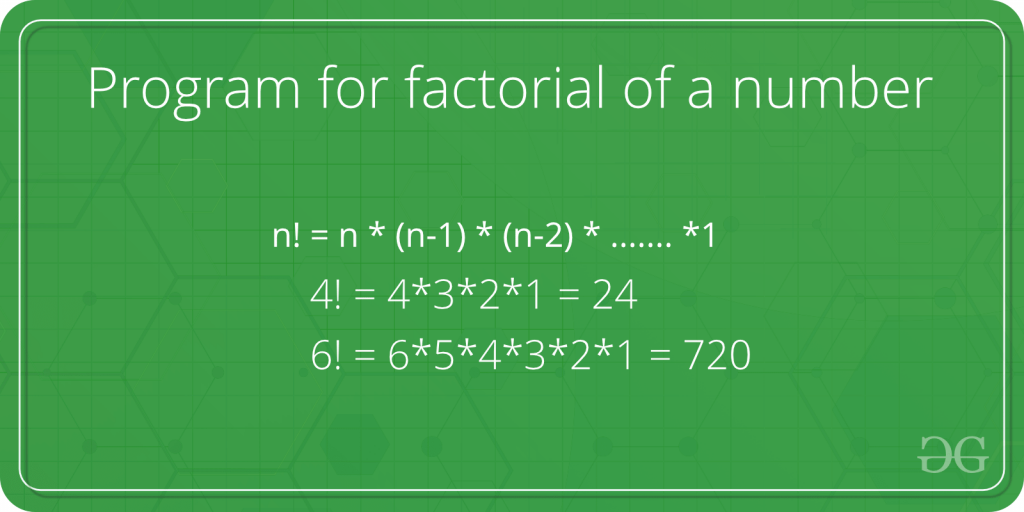Open In App

# Factorial Program in C

A factorial is the product of all the natural numbers less than or equal to the given number n. For Example, the factorial of 6 is 6 * 5 * 4 * 3 * 2 * 1 which is 720. In this article, we will explore a few programs to find the factorial of a number in C.We can write the factorial program in C using two methods:

1. Using Loops
2. Using Recursion

## 1. Factorial Program using for Loop

In this method, we use a loop that runs (n – 1) times to get the product of all numbers starting from 1 to n. The below C program use for loop to find the factorial.

## C

 `// C program to implement the above approach``#include ` `// Function to find factorial of given number``unsigned ``int` `factorial(unsigned ``int` `n)``{``    ``int` `result = 1, i;` `    ``// loop from 2 to n to get the factorial``    ``for` `(i = 2; i <= n; i++) {``        ``result *= i;``    ``}` `    ``return` `result;``}` `// Driver code``int` `main()``{``    ``int` `num = 5;``    ``printf``(``"Factorial of %d is %d"``, num, factorial(num));``    ``return` `0;``}`

Output

```Factorial of 5 is 120

```

Time Complexity: O(n)
Auxiliary Space: O(1)

## 2. Factorial Program using Recursion

In this method, we find the factorial in C using a recursive function. This recursive function will call itself n number of times and each time, the value of n will be decreased by 1. The recursion will stop when n = 1.

## C

 `// C program to find factorial of given number``#include ` `// Function to find factorial of given number``unsigned ``int` `factorial(unsigned ``int` `n)``{``    ``if` `(n == 1) {``      ``return` `1;``    ``}``  ` `    ``return` `n * factorial(n - 1);``}` `// Driver code``int` `main()``{``    ``int` `num = 5;``    ``printf``(``"Factorial of %d is %d"``, num, factorial(num));``    ``return` `0;``}`

Output

```Factorial of 5 is 120

```

Time Complexity: O(n)
Auxiliary Space: O(n)

For more programs to find the factorial, refer to the article – Program for factorial of a number